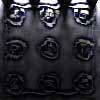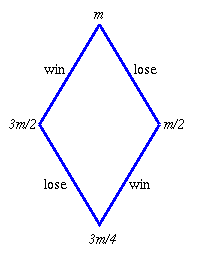#### You may also like### Fixing the Odds

You have two bags, four red balls and four white balls. You must put all the balls in the bags although you are allowed to have one bag empty. How should you distribute the balls between the two bags so as to make the probability of choosing a red ball as small as possible and what will the probability be in that case?### Scratch Cards

To win on a scratch card you have to uncover three numbers that add up to more than fifteen. What is the probability of winning a prize?### In a Box

Chris and Jo put two red and four blue ribbons in a box. They each pick a ribbon from the box without looking. Jo wins if the two ribbons are the same colour. Is the game fair?

# Win or Lose?

##### Age 14 to 16Challenge Level

The gambler will have less money than he started with.

Suppose the amount of money before a game is $m$, then:

$m \to 3m/2$ for a win and $m\to m/2$ after losing a game.Values of n Amount after 2n games: n wins, n losses
1 $3m/4$
2 $m \times 1/2 \times3/2 \times1/2 \times3/2 = (3/4)^2 m$
3 $m \times1/2 \times3/2 \times1/2 \times3/2 \times1/2 \times3/2 = (3/4)^3 m$

After $n$ wins and $n$ losses he will have $(3/4)^n$ times the money he started with, irrespective of the order in which his wins and losses occur. Eventually he will run out of money as what he has left will be smaller than the smallest coin in circulation.

The diagram was suggested by Roderick and Michael of Simon Langton Boys' Grammar School Canterbury who pointed out that if the gambler went on indefinitely he would, in theory, end up with an infinitely small amount which would be represented by nothing.